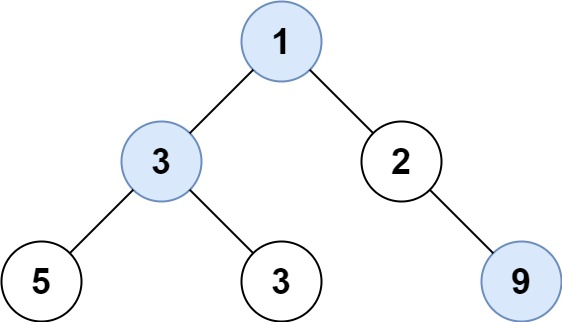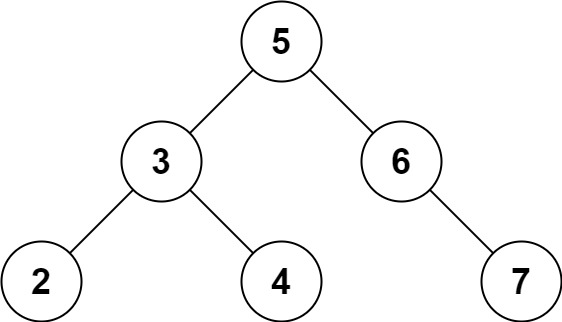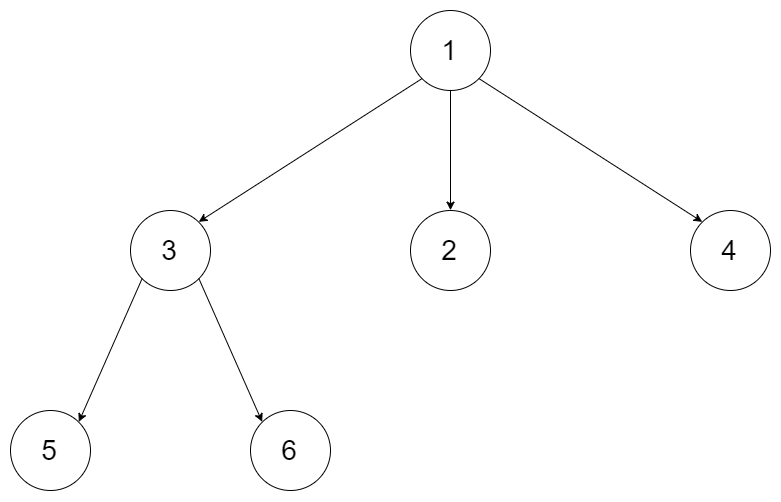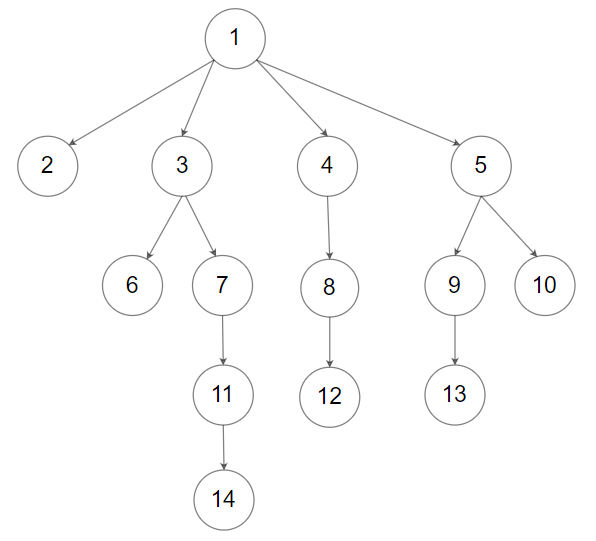## C# || Parallel Courses III – How To Find Minimum Number Months To Complete All Courses Using C#The following is a module with functions which demonstrates how to find the minimum number of months needed to complete all courses using C#.

1. Minimum Time – Problem Statement

You are given an integer n, which indicates that there are n courses labeled from 1 to n. You are also given a 2D integer array relations where relations[j] = [prevCoursej, nextCoursej] denotes that course prevCoursej has to be completed before course nextCoursej (prerequisite relationship). Furthermore, you are given a 0-indexed integer array time where time[i] denotes how many months it takes to complete the (i+1)th course.

You must find the minimum number of months needed to complete all the courses following these rules:

• You may start taking a course at any time if the prerequisites are met.
• Any number of courses can be taken at the same time.

Return the minimum number of months needed to complete all the courses.

Note: The test cases are generated such that it is possible to complete every course (i.e., the graph is a directed acyclic graph).

Example 1:``` Input: n = 3, relations = [[1,3],[2,3]], time = [3,2,5] Output: 8 Explanation: The figure above represents the given graph and the time required to complete each course. We start course 1 and course 2 simultaneously at month 0. Course 1 takes 3 months and course 2 takes 2 months to complete respectively. Thus, the earliest time we can start course 3 is at month 3, and the total time required is 3 + 5 = 8 months. ```

Example 2:``` Input: n = 5, relations = [[1,5],[2,5],[3,5],[3,4],[4,5]], time = [1,2,3,4,5] Output: 12 Explanation: The figure above represents the given graph and the time required to complete each course. You can start courses 1, 2, and 3 at month 0. You can complete them after 1, 2, and 3 months respectively. Course 4 can be taken only after course 3 is completed, i.e., after 3 months. It is completed after 3 + 4 = 7 months. Course 5 can be taken only after courses 1, 2, 3, and 4 have been completed, i.e., after max(1,2,3,7) = 7 months. Thus, the minimum time needed to complete all the courses is 7 + 5 = 12 months. ```

2. Minimum Time – Solution

The following is a solution which demonstrates how to find the minimum number of months needed to complete all courses.

``` 2. Minimum Time - Solution C# // ============================================================================ // Author: Kenneth Perkins // Date: Nov 1, 2023 // Taken From: http://programmingnotes.org/ // File: Solution.cs // Description: Demonstrates how to find the minimum months complete courses // ============================================================================ public class Solution { public int MinimumTime(int n, int[][] relations, int[] time) { var graph = new Dictionary<int, List<int>>(); for (int i = 0; i < n; i++) { graph[i] = new List<int>(); } int[] indegree = new int[n]; foreach (int[] edge in relations) { int x = edge - 1; int y = edge - 1; graph[x].Add(y); indegree[y]++; } var queue = new Queue<int>(); int[] maxTime = new int[n]; for (int node = 0; node < n; node++) { if (indegree[node] == 0) { queue.Enqueue(node); maxTime[node] = time[node]; } } while (queue.Count > 0) { int node = queue.Dequeue(); foreach (int neighbor in graph[node]) { maxTime[neighbor] = Math.Max(maxTime[neighbor], maxTime[node] + time[neighbor]); indegree[neighbor]--; if (indegree[neighbor] == 0) { queue.Enqueue(neighbor); } } } int ans = 0; for (int node = 0; node < n; node++) { ans = Math.Max(ans, maxTime[node]); } return ans; } }// http://programmingnotes.org/ 123456789101112131415161718192021222324252627282930313233343536373839404142434445464748495051 // ============================================================================//    Author: Kenneth Perkins//    Date:   Nov 1, 2023//    Taken From: http://programmingnotes.org///    File:  Solution.cs//    Description: Demonstrates how to find the minimum months complete courses// ============================================================================public class Solution {    public int MinimumTime(int n, int[][] relations, int[] time) {        var graph = new Dictionary<int, List<int>>();        for (int i = 0; i < n; i++) {            graph[i] = new List<int>();        }         int[] indegree = new int[n];        foreach (int[] edge in relations) {            int x = edge - 1;            int y = edge - 1;            graph[x].Add(y);            indegree[y]++;        }         var queue = new Queue<int>();        int[] maxTime = new int[n];         for (int node = 0; node < n; node++) {            if (indegree[node] == 0) {                queue.Enqueue(node);                maxTime[node] = time[node];            }        }         while (queue.Count > 0) {            int node = queue.Dequeue();            foreach (int neighbor in graph[node]) {                maxTime[neighbor] = Math.Max(maxTime[neighbor], maxTime[node] + time[neighbor]);                indegree[neighbor]--;                if (indegree[neighbor] == 0) {                    queue.Enqueue(neighbor);                }            }        }         int ans = 0;        for (int node = 0; node < n; node++) {            ans = Math.Max(ans, maxTime[node]);        }         return ans;    }}// http://programmingnotes.org/ ```

QUICK NOTES:
The highlighted lines are sections of interest to look out for.

The code is heavily commented, so no further insight is necessary. If you have any questions, feel free to leave a comment below.

Once compiled, you should get this as your output for the example cases:

``` 8 12 ```

## C# || How To Find Largest Value In Each Binary Tree Row Using C#The following is a module with functions which demonstrates how to find the largest value in each binary tree row using C#.

1. Largest Values – Problem Statement

Given the root of a binary tree, return an array of the largest value in each row of the tree (0-indexed).

Example 1:``` Input: root = [1,3,2,5,3,null,9] Output: [1,3,9] ```

Example 2:

``` Input: root = [1,2,3] Output: [1,3] ```

2. Largest Values – Solution

The following is a solution which demonstrates how to find the largest value in each binary tree row.

``` 2. Largest Values - Solution C# // ============================================================================ // Author: Kenneth Perkins // Date: Oct 28, 2023 // Taken From: http://programmingnotes.org/ // File: Solution.cs // Description: Demonstrates how to find the largest value binary tree row // ============================================================================ /** * Definition for a binary tree node. * public class TreeNode { * public int val; * public TreeNode left; * public TreeNode right; * public TreeNode(int val=0, TreeNode left=null, TreeNode right=null) { * this.val = val; * this.left = left; * this.right = right; * } * } */ public class Solution { public IList<int> LargestValues(TreeNode root) { if (root == null) { return new List<int>(); } var ans = new List<int>(); var queue = new Queue<TreeNode>(); queue.Enqueue(root); while (queue.Count > 0) { int currMax = int.MinValue; for (int i = queue.Count -1; i >= 0; --i) { var node = queue.Dequeue(); currMax = Math.Max(currMax, node.val); if (node.left != null) { queue.Enqueue(node.left); } if (node.right != null) { queue.Enqueue(node.right); } } ans.Add(currMax); } return ans; } }// http://programmingnotes.org/ 123456789101112131415161718192021222324252627282930313233343536373839404142434445464748 // ============================================================================//    Author: Kenneth Perkins//    Date:   Oct 28, 2023//    Taken From: http://programmingnotes.org///    File:  Solution.cs//    Description: Demonstrates how to find the largest value binary tree row// ============================================================================/** * Definition for a binary tree node. * public class TreeNode { *     public int val; *     public TreeNode left; *     public TreeNode right; *     public TreeNode(int val=0, TreeNode left=null, TreeNode right=null) { *         this.val = val; *         this.left = left; *         this.right = right; *     } * } */public class Solution {    public IList<int> LargestValues(TreeNode root) {        if (root == null) {            return new List<int>();        }         var ans = new List<int>();        var queue = new Queue<TreeNode>();        queue.Enqueue(root);         while (queue.Count > 0) {            int currMax = int.MinValue;            for (int i = queue.Count -1; i >= 0; --i) {                var node = queue.Dequeue();                currMax = Math.Max(currMax, node.val);                 if (node.left != null) {                    queue.Enqueue(node.left);                }                if (node.right != null) {                    queue.Enqueue(node.right);                }            }            ans.Add(currMax);        }        return ans;    }}// http://programmingnotes.org/ ```

QUICK NOTES:
The highlighted lines are sections of interest to look out for.

The code is heavily commented, so no further insight is necessary. If you have any questions, feel free to leave a comment below.

Once compiled, you should get this as your output for the example cases:

``` [1,3,9] [1,3] ```

## C# || Two Sum IV – How To Get Two Numbers In Binary Search Tree Equal To Target Value Using C#The following is a module with functions which demonstrates how to get two numbers in a binary search tree equal to target value using C#.

1. Find Target – Problem Statement

Given the root of a Binary Search Tree and a target number k, return true if there exist two elements in the BST such that their sum is equal to the given target.

Example 1:``` Input: root = [5,3,6,2,4,null,7], k = 9 Output: true ```

Example 2:``` Input: root = [5,3,6,2,4,null,7], k = 28 Output: false ```

2. Find Target – Solution

The following are two solutions which demonstrates how to get two numbers in a binary search tree equal to target value.

Both solutions use a set to keep track of the items already seen.

Each time a new node is encountered, we subtract the target value from the current node value. If the difference amount from subtracting the two numbers exists in the set, a 2 sum combination exists in the tree

1. Recursive

The following solution uses Depth First Search when looking for the target value.

``` 2. Find Target - Solution - Recursive C# // ============================================================================ // Author: Kenneth Perkins // Date: Oct 9, 2022 // Taken From: http://programmingnotes.org/ // File: Solution.cs // Description: Demonstrates how to get two numbers equal to target value // ============================================================================ public class Solution { public bool FindTarget(TreeNode root, int k) { return Traverse(root, k, new HashSet<int>()); } private bool Traverse(TreeNode node, int k, HashSet<int> seen) { if (node == null) { return false; } // Get remaining value var remaining = k - node.val; // Check if remaining value has been seen if (seen.Contains(remaining)) { return true; } // Add current node value to items seen seen.Add(node.val); // Keep traversing left and right looking for 2 sum return Traverse(node.left, k, seen) || Traverse(node.right, k, seen); } }// http://programmingnotes.org/ 123456789101112131415161718192021222324252627282930 // ============================================================================//    Author: Kenneth Perkins//    Date:   Oct 9, 2022//    Taken From: http://programmingnotes.org///    File:  Solution.cs//    Description: Demonstrates how to get two numbers equal to target value// ============================================================================public class Solution {    public bool FindTarget(TreeNode root, int k) {        return Traverse(root, k, new HashSet<int>());    }     private bool Traverse(TreeNode node, int k, HashSet<int> seen) {        if (node == null) {            return false;        }        // Get remaining value        var remaining = k - node.val;         // Check if remaining value has been seen        if (seen.Contains(remaining)) {            return true;        }        // Add current node value to items seen        seen.Add(node.val);         // Keep traversing left and right looking for 2 sum        return Traverse(node.left, k, seen) || Traverse(node.right, k, seen);    }}// http://programmingnotes.org/ ```

2. Iterative

The following solution uses Breadth First Search when looking for the target value.

``` 2. Find Target - Solution - Iterative C# // ============================================================================ // Author: Kenneth Perkins // Date: Oct 9, 2022 // Taken From: http://programmingnotes.org/ // File: Solution.cs // Description: Demonstrates how to get two numbers equal to target value // ============================================================================ public class Solution { public bool FindTarget(TreeNode root, int k) { if (root == null) { return false; } // Declare stack var stack = new Stack<TreeNode>(); // Keep track of values seen var seen = new HashSet<int>(); // Add root to stack stack.Push(root); // Loop through items on the stack while (stack.Count > 0) { // Get current node var node = stack.Pop(); // Get remaining value var remaining = k - node.val; // Check if remaining value has been seen if (seen.Contains(remaining)) { return true; } else { // Keep traversing left and right looking for 2 sum if (node.left != null) { stack.Push(node.left); } if (node.right != null) { stack.Push(node.right); } } // Add current node value to items seen seen.Add(node.val); } return false; } }// http://programmingnotes.org/ 1234567891011121314151617181920212223242526272829303132333435363738394041424344454647 // ============================================================================//    Author: Kenneth Perkins//    Date:   Oct 9, 2022//    Taken From: http://programmingnotes.org///    File:  Solution.cs//    Description: Demonstrates how to get two numbers equal to target value// ============================================================================public class Solution {    public bool FindTarget(TreeNode root, int k) {        if (root == null) {            return false;        }        // Declare stack        var stack = new Stack<TreeNode>();         // Keep track of values seen        var seen = new HashSet<int>();         // Add root to stack        stack.Push(root);         // Loop through items on the stack        while (stack.Count > 0) {            // Get current node            var node = stack.Pop();             // Get remaining value            var remaining = k - node.val;             // Check if remaining value has been seen            if (seen.Contains(remaining)) {                return true;            } else {                // Keep traversing left and right looking for 2 sum                if (node.left != null) {                    stack.Push(node.left);                }                if (node.right != null) {                    stack.Push(node.right);                }            }            // Add current node value to items seen            seen.Add(node.val);        }        return false;    }}// http://programmingnotes.org/ ```

QUICK NOTES:
The highlighted lines are sections of interest to look out for.

The code is heavily commented, so no further insight is necessary. If you have any questions, feel free to leave a comment below.

Once compiled, you should get this as your output for the example cases:

``` true false ```

## C# || How To Traverse N-ary Tree Level Order Using C#The following is a module with functions which demonstrates how to traverse a N-ary Tree level order using C#.

1. Level Order – Problem Statement

Given an n-ary tree, return the level order traversal of its nodes’ values.

Nary-Tree input serialization is represented in their level order traversal, each group of children is separated by the null value (See examples).

Example 1:``` Input: root = [1,null,3,2,4,null,5,6] Output: [,[3,2,4],[5,6]] ```

Example 2:``` Input: root = [1,null,2,3,4,5,null,null,6,7,null,8,null,9,10,null,null,11,null,12,null,13,null,null,14] Output: [,[2,3,4,5],[6,7,8,9,10],[11,12,13],] ```

2. Level Order – Solution

The following is a solution which demonstrates how to traverse a N-ary Tree level order.

This solution uses Breadth First Search to explore items at each level.

``` 2. Level Order - Solution C# // ============================================================================ // Author: Kenneth Perkins // Date: Sep 5, 2022 // Taken From: http://programmingnotes.org/ // File: Solution.cs // Description: Demonstrates how to traverse a N-ary Tree Level Order // ============================================================================ /* // Definition for a Node. public class Node { public int val; public IList<Node> children; public Node() {} public Node(int _val) { val = _val; } public Node(int _val, IList<Node> _children) { val = _val; children = _children; } } */ public class Solution { public IList<IList<int>> LevelOrder(Node root) { var result = new List<IList<int>>(); if (root == null) { return result; } var queue = new Queue<Node>(); queue.Enqueue(root); while (queue.Count > 0) { var currentLevel = new List<int>(); for (int count = queue.Count - 1; count >= 0 ; --count) { var currentNode = queue.Dequeue(); currentLevel.Add(currentNode.val); foreach (var child in currentNode.children) { queue.Enqueue(child); } } result.Add(currentLevel); } return result; } }// http://programmingnotes.org/ 1234567891011121314151617181920212223242526272829303132333435363738394041424344454647484950 // ============================================================================//    Author: Kenneth Perkins//    Date:   Sep 5, 2022//    Taken From: http://programmingnotes.org///    File:  Solution.cs//    Description: Demonstrates how to traverse a N-ary Tree Level Order// ============================================================================/*// Definition for a Node.public class Node {    public int val;    public IList<Node> children;     public Node() {}     public Node(int _val) {        val = _val;    }     public Node(int _val, IList<Node> _children) {        val = _val;        children = _children;    }}*/public class Solution {    public IList<IList<int>> LevelOrder(Node root) {        var result = new List<IList<int>>();        if (root == null) {           return result;        }         var queue = new Queue<Node>();        queue.Enqueue(root);         while (queue.Count > 0) {            var currentLevel = new List<int>();            for (int count = queue.Count - 1; count >= 0 ; --count) {                var currentNode = queue.Dequeue();                currentLevel.Add(currentNode.val);                foreach (var child in currentNode.children) {                    queue.Enqueue(child);                }            }            result.Add(currentLevel);        }         return result;    }}// http://programmingnotes.org/ ```

QUICK NOTES:
The highlighted lines are sections of interest to look out for.

The code is heavily commented, so no further insight is necessary. If you have any questions, feel free to leave a comment below.

Once compiled, you should get this as your output for the example cases:

``` [,[3,2,4],[5,6]] [,[2,3,4,5],[6,7,8,9,10],[11,12,13],] ```